# 7 Results

View
Selected filters:
• MCCRS.Math.Content.5.G.B.4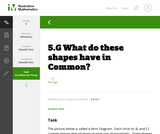Unrestricted Use
CC BY
Rating

This is a task from the Illustrative Mathematics website that is one part of a complete illustration of the standard to which it is aligned. Each task has at least one solution and some commentary that addresses important aspects of the task and its potential use.

Subject:
Geometry
Material Type:
Activity/Lab
Provider:
Illustrative Mathematics
Provider Set:
Illustrative Mathematics
Author:
Illustrative Mathematics
08/06/2015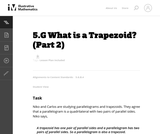Unrestricted Use
CC BY
Rating

This is a task from the Illustrative Mathematics website that is one part of a complete illustration of the standard to which it is aligned. Each task has at least one solution and some commentary that addresses important asects of the task and its potential use. Here are the first few lines of the commentary for this task: Niko and Carlos are studying parallelograms and trapezoids. They agree that a parallelogram is a quadrilateral with 2 pairs of parallel sides. Niko say...

Subject:
Mathematics
Material Type:
Activity/Lab
Provider:
Illustrative Mathematics
Provider Set:
Illustrative Mathematics
Author:
Illustrative Mathematics
05/20/2013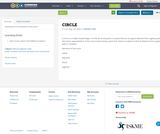Conditional Remix & Share Permitted
CC BY-NC
Rating

meaning of circle
elements of the circle

Subject:
Education
Mathematics
Geometry
Material Type:
Assessment
Homework/Assignment
Author:
VAIBHAVI TANK
08/26/2019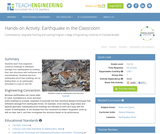Educational Use
Rating

Students learn how engineers construct buildings to withstand damage from earthquakes by building their own structures with toothpicks and marshmallows. Students test how earthquake-proof their buildings are by testing them on an earthquake simulated in a pan of Jell-O(TM).

Subject:
Engineering
Geology
Material Type:
Activity/Lab
Provider:
TeachEngineering
Provider Set:
TeachEngineering
Author:
Janet Yowell
Jessica Todd
Malinda Schaefer Zarske
Melissa Straten
10/14/2015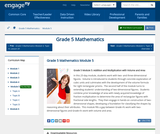Conditional Remix & Share Permitted
CC BY-NC-SA
Rating

In this 25-day module, students work with two- and three-dimensional figures.  Volume is introduced to students through concrete exploration of cubic units and culminates with the development of the volume formula for right rectangular prisms.  The second half of the module turns to extending students understanding of two-dimensional figures.  Students combine prior knowledge of area with newly acquired knowledge of fraction multiplication to determine the area of rectangular figures with fractional side lengths.  They then engage in hands-on construction of two-dimensional shapes, developing a foundation for classifying the shapes by reasoning about their attributes.  This module fills a gap between Grade 4s work with two-dimensional figures and Grade 6s work with volume and area.

Subject:
Geometry
Material Type:
Module
Provider:
New York State Education Department
Provider Set:
EngageNY
01/17/2014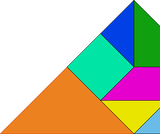Unrestricted Use
CC BY
Rating

Students learn about the history of tangrams.  They will learn about each piece in the tangram puzzle and analyze the shapes to complete geometric puzzles and mathematics problems.

Subject:
Mathematics
Geometry
Material Type:
Lesson Plan
Author:
Lynn Ann Wiscount
Vince Mariner
Erin Halovanic
07/13/2020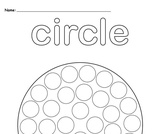Unrestricted Use
CC BY
Rating

A circle is a simple closed shape. It is the set of all points in a plane that are at a given distance from a given point, the centre; equivalently it is the curve traced out by a point that moves in a plane so that its distance from a given point is constant.elements of the circleradiuschorddiametersegment

Subject:
Education
Mathematics
Material Type:
Lesson Plan
Author:
VAIBHAVI TANK